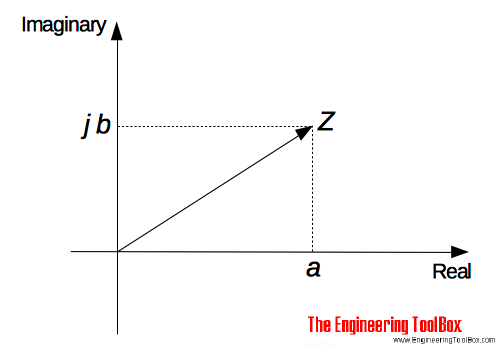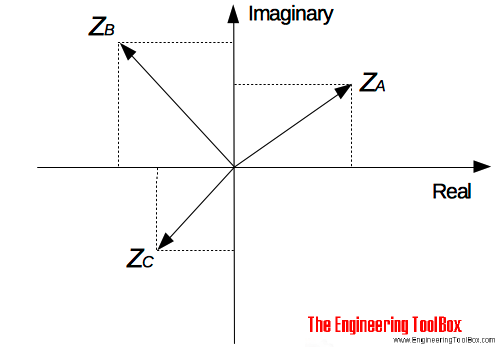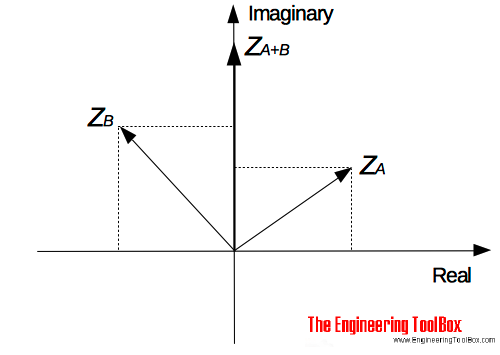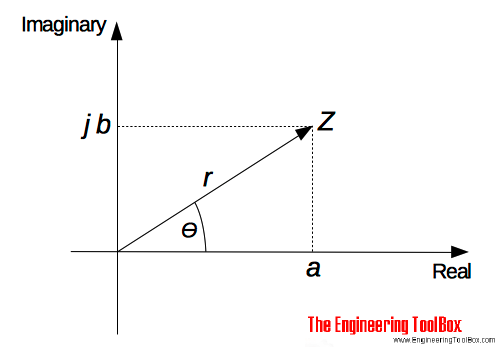Engineering ToolBox - Resources, Tools and Basic Information for Engineering and Design of Technical Applications!

# Complex Numbers

## Complex numbers are used in alternating current theory and mechanical vector analysis.

There are two main forms of complex numbers

• Cartesian
• Polar

### Complex numbers on the Cartesian form

A complex number consists of a real part and an imaginary part and can be expressed on the Cartesian form as

Z = a + j b                         (1)

where

Z = complex number

a = real part

j b = imaginary part (it is common to use i instead of j)

A complex number can be represented in a Cartesian axis diagram with an real and an imaginary axis - also called the Argand diagram:#### Example - Complex numbers on the Cartesian form

The complex numbers

ZA = 3 + j 2                              (2a)

ZB = -3 + j 3                             (2b)

ZC = -2 - j 2                             (2c)

can be represented in the Argand diagram:#### Addition and Subtraction of Complex numbers

Complex numbers are added/subtracted by adding/subtracting the separately the real parts and the imaginary parts of the number.

##### Example - Adding two Complex numbers

ZA = 3 + j 2

ZB = -3 + j 3

Z(A+B) = (3 + (-3)) + (j 2 + j 3)

= j 5### Complex numbers on the Polar form

A complex number on the polar form can be expressed as

Z = r (cosθ + j sinθ)                   (3)

where

r = modulus (or magnitude) of Z - and is written as "mod Z" or |Z|

θ = argument (or amplitude) of Z - and is written as "arg Z"r can be determined using Pythagoras' theorem

r = (a2 + b2)1/2                          (4)

θ can be determined by trigonometry

θ = tan-1(b / a)                          (5)

(3) can also be expressed as

Z = r ej θ                    (6)

As we can se from (1), (3) and (6) - a complex number can be written in three different ways.

#### Example - Complex number on the Polar form

The complex number

Za = 3 + j 2

can be expressed on the polar form by calculating the modulus and the argument.

The "modulus" can be calculated by using eq. (4):

r = (32 + 22)1/2

=  3.606

The "argument" can be calculated by using eq. (5):

θ = tan-1(2 / 3)

= 33.69o

The complex number on polar form (3):

Za = 3.606 (cos(33.69) + j sin(33.69))

Or alternatively (6)

Za = 3.606 ej 33.69

### Adding or Subtraction of Complex Numbers

Za = a + j b

Zb = c + j d

Za + Zb = (a + j b) + (c + j d)

= (a + c) + j(b + d)                            (6)

or alternative

Za = ra (cosθa + j sinθa)

Zb = rb (cosθb + j sinθb)

Za + Zbra (cosθa + j sinθa) + rb (cosθb + j sinθb)

= (ra cosθa + rb cosθb) + j (ra sinθarb sinθb)                            (6b)

or alternatively

Za = ra ejθa

Zb rb ejθb

Za + Zb = ra ejθa + rb ejθb

= (ra cosθa + rb cosθb) + j (ra sinθarb sinθb)                            (6c)

##### Example - Adding Complex Numbers

Za = 3 + j 2

Zb = 5 - j 4

Za + Zb = (3 + j 2) + (5 - j 4)

= (3 + 5) + j(2 + (-4))

= 8 - j 2

##### Example - Adding Complex Numbers

Za = 3 (cos 35 + j sin 35)

Zb = 2 (cos 15 + j sin 15)

Za + Zb = (3 cos 35+ 2 cos 15) + j (3 sin 35 +  2 sin 15)

= 4.38 - j 2.2

#### Subtracting Complex Numbers

Za = a + j b

Zb = c + j d

Za - Zb = (a + j b) - (c + j d)

= (a - c) + j(b - d)                           (7)

##### Example - Subtracting Complex Numbers

Za = 3 (cos 35 + j sin 35)

Zb = 2 (cos 15 + j sin 15)

Za - Zb = 3 (cos 35 + j sin 35) - 2 (cos 15 + j sin 15)

= (3 cos 35 - 2 cos 15) + j (3 sin 35 - 2 sin 15)

=  0.52 + j 1.2

### Multiplication of Complex Numbers

Za = a + j b

Zb = c + j d

Za Zb = (a + j b) (c + j d)

= a c + a (j d) + (j b) c + (j b) (j d)

= a c + j a d + j b c + j2 b d                           (8)

Since j2 = -1  - (8) can be transformed to

Za Zb = (a + j b) (c + j d)

= (a c - b d) + j (a d + b c)                          (8b)

##### Example - Multiplying Complex Numbers

Za3 + j 2

Zb5 - j 4

Za Zb = (3 + j 2) (5 - j 4)

= (3 5 - 2 (-4)) + j(3 (-4) + 2 5)

= 23 - j 2

### Complex Conjugate

The complex conjugate of (a + jb) is (a - jb).

Multiplying a complex number with its complex conjugate results in a real number like

Za = a + jb

Za* = a - jb

Za Za* = (a + jb) (a - jb)

= a2 - j a b + j a b - j2 b2

= a2 - (- b2)

= a2 + b2                                 (9)

##### Example - Multiplying a Complex Number with its Conjugate

Za3 + j 2

Za*3 - j 2

Za Za* = (3 + j 2) (3 - j 2)

= 32 + 22

= 13

### Division of Complex Numbers

Division of complex numbers can be done with the help of the denominators conjugate:

Za = a + jb

Zbc + j d

Za / Zb = (a + j b) / (c + j d)

= ((a + j b) / (c + j d)) ((c - j d) / (c - j d))

= (a c +  j a d + j b c + j2 b d) / (c2 + d2)                                  (10)

Multiplying both the nominator and the denominator with the conjugate of the denominator is called rationalizing.

## Related Topics

• Mathematics - Mathematical rules and laws - numbers, areas, volumes, exponents, trigonometric functions and more.

## Engineering ToolBox - SketchUp Extension - Online 3D modeling!

Add standard and customized parametric components - like flange beams, lumbers, piping, stairs and more - to your Sketchup model with the Engineering ToolBox - SketchUp Extension - enabled for use with the amazing, fun and free SketchUp Make and SketchUp Pro .Add the Engineering ToolBox extension to your SketchUp from the SketchUp Pro Sketchup Extension Warehouse!

Translate

## Privacy

We don't collect information from our users. Only emails and answers are saved in our archive. Cookies are only used in the browser to improve user experience.

Some of our calculators and applications let you save application data to your local computer. These applications will - due to browser restrictions - send data between your browser and our server. We don't save this data.

## Citation

• Engineering ToolBox, (2016). Complex Numbers. [online] Available at: https://www.engineeringtoolbox.com/complex-numbers-d_1921.html [Accessed Day Mo. Year].

Modify access date.

. .

#### Scientific Online Calculator6 24